### Day 23 - Factoring and Distributive Property - 02.10.15

• Unit 3 Test next Friday, February 20th!

Bell Ringer

1. Find the prime factorization of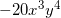1.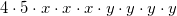2.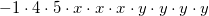3.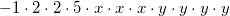4.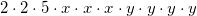5. none of the above

2. Find the GCF of the following set of monomials: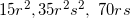1. 5

2. 15r

3. 5r

4. 5s

5. none of the above

3. Find the GCF for each term in the following polynomial: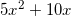1. 5

2. 10

3. 5x

4. 5x2

5. none of the above

4. Factor the following polynomial by reverse distributing:1.2.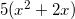3.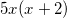4.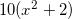5. none of the above

5. Solve the following equation: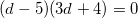. Use this video for help...

1. 5

2. -5

3.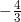4. both a and c

5. none of the above

Review
• Prerequisites
• Prime Numbers
• Composite Numbers
• Factor
• Distributive Property
• Factoring (video 1) (video 2)
• Classifying Numbers as Prime or Composite
• Prime Factorization of a Positive Integer
• Prime Factorization of a Negative Integer
• Prime Factorization of a Monomial
• Finding the GCF of an Integer
• Finding the GCF of a Monomial
• Apply Factoring to Solve Problems

Lesson

Exit Ticket
• Posted on board at the end of the block
Lesson Objective(s)
• How can polynomials be factoring using Distributive Property?
Skills
• Factor polynomials using the Distributive Property
• Factor by grouping
• Solve quadratic equations of the following form:

#### In-Class Help Requests

Standard(s)
• CC.9-12.A.SSE.1a Interpret parts of an expression, such as terms, factors, and coefficients.

Mathematical Practice(s)
• #1 - Make sense of problems and persevere in solving them
• #2 - Reason abstractly and quantitatively
• #7 - Look for and make use of structure

Past Checkpoints

Unit Skills
1. Classifying Numbers as Prime or Composite
2. Prime Factorization of a Positive Integer
3. Prime Factorization of a Negative Integer
4. Prime Factorization of a Monomial
5. Finding the GCF of an Integer
6. Finding the GCF of a Monomial
7. Factor Applications